$$\newcommand{\id}{\mathrm{id}}$$ $$\newcommand{\Span}{\mathrm{span}}$$ $$\newcommand{\kernel}{\mathrm{null}\,}$$ $$\newcommand{\range}{\mathrm{range}\,}$$ $$\newcommand{\RealPart}{\mathrm{Re}}$$ $$\newcommand{\ImaginaryPart}{\mathrm{Im}}$$ $$\newcommand{\Argument}{\mathrm{Arg}}$$ $$\newcommand{\norm}{\| #1 \|}$$ $$\newcommand{\inner}{\langle #1, #2 \rangle}$$ $$\newcommand{\Span}{\mathrm{span}}$$

# 6.2: Solve Quadratic Equations by Completing the Square

•• OpenStax
• OpenStax
$$\newcommand{\vecs}{\overset { \rightharpoonup} {\mathbf{#1}} }$$ $$\newcommand{\vecd}{\overset{-\!-\!\rightharpoonup}{\vphantom{a}\smash {#1}}}$$$$\newcommand{\id}{\mathrm{id}}$$ $$\newcommand{\Span}{\mathrm{span}}$$ $$\newcommand{\kernel}{\mathrm{null}\,}$$ $$\newcommand{\range}{\mathrm{range}\,}$$ $$\newcommand{\RealPart}{\mathrm{Re}}$$ $$\newcommand{\ImaginaryPart}{\mathrm{Im}}$$ $$\newcommand{\Argument}{\mathrm{Arg}}$$ $$\newcommand{\norm}{\| #1 \|}$$ $$\newcommand{\inner}{\langle #1, #2 \rangle}$$ $$\newcommand{\Span}{\mathrm{span}}$$ $$\newcommand{\id}{\mathrm{id}}$$ $$\newcommand{\Span}{\mathrm{span}}$$ $$\newcommand{\kernel}{\mathrm{null}\,}$$ $$\newcommand{\range}{\mathrm{range}\,}$$ $$\newcommand{\RealPart}{\mathrm{Re}}$$ $$\newcommand{\ImaginaryPart}{\mathrm{Im}}$$ $$\newcommand{\Argument}{\mathrm{Arg}}$$ $$\newcommand{\norm}{\| #1 \|}$$ $$\newcommand{\inner}{\langle #1, #2 \rangle}$$ $$\newcommand{\Span}{\mathrm{span}}$$

##### Learning Objectives

By the end of this section, you will be able to:

• Complete the square of a binomial expression
• Solve quadratic equations of the form $$x^{2}+bx+c=0$$ by completing the square
• Solve quadratic equations of the form $$ax^{2}+bx+c=0$$ by completing the square

Before you get started, take this readiness quiz.

1. Expand: $$(x+9)^{2}$$.
If you missed this problem, review Example 5.32.
2. Factor $$y^{2}-14 y+49$$.
If you missed this problem, review Example 6.9.
3. Factor $$5 n^{2}+40 n+80$$.
If you missed this problem, review Example 6.14.

So far we have solved quadratic equations by factoring and using the Square Root Property. In this section, we will solve quadratic equations by a process called completing the square, which is important for our work on conics later.

## Complete the Square of a Binomial Expression

In the last section, we were able to use the Square Root Property to solve the equation $$(y-7)^{2}=12$$ because the left side was a perfect square.

\begin{aligned}(y-7)^{2} &=12 \\ y-7 &=\pm \sqrt{12} \\ y-7 &=\pm 2 \sqrt{3} \\ y &=7 \pm 2 \sqrt{3} \end{aligned}

We also solved an equation in which the left side was a perfect square trinomial, but we had to rewrite it the form $$(x−k)^{2}$$ in order to use the Square Root Property.

\begin{aligned} x^{2}-10 x+25 &=18 \\(x-5)^{2} &=18 \end{aligned}

What happens if the variable is not part of a perfect square? Can we use algebra to make a perfect square?

Let’s look at two examples to help us recognize the patterns.

$$\begin{array}{cc}{(x+9)^{2}} & {(y-7)^{2}} \\ {(x+9 )(x+9)} & {(y-7)(y-7)} \\ {x^{2}+9 x+9 x+81} & {y^{2}-7 y-7 y+49} \\ {x^{2}+18 x+81} & {y^{2}-14 y+49}\end{array}$$

We restate the patterns here for reference.

##### Definition $$\PageIndex{1}$$: Binomial Squares Pattern

If $$a$$ and $$b$$ are real numbers,

We can use this pattern to “make” a perfect square.

We will start with the expression $$x^{2}+6 x$$. Since there is a plus sign between the two terms, we will use the $$(a+b)^{2}$$ pattern, $$a^{2}+2 a b+b^{2}=(a+b)^{2}$$.

We ultimately need to find the last term of this trinomial that will make it a perfect square trinomial. To do that we will need to find $$b$$. But first we start with determining $$a$$. Notice that the first term of $$x^{2}+6x$$ is a square, $$x^{2}$$. This tells us that $$a=x$$.

What number, $$b$$, when multiplied with $$2x$$ gives $$6x$$? It would have to be $$3$$, which is $$\frac{1}{2}(6)$$. So $$b=3$$.

Now to complete the perfect square trinomial, we will find the last term by squaring $$b$$, which is $$3^{2}=9$$.

We can now factor.

So we found that adding $$9$$ to $$x^{2}+6 x$$ ‘completes the square’, and we write it as $$(x+3)^{2}$$.

##### Howto: Complete a Square of $$x^{2}+bx$$
1. Identify $$b$$, the coefficient of $$x$$.
2. Find $$\left(\frac{1}{2} b\right)^{2}$$, the number to complete the square.
3. Add the$$\left(\frac{1}{2} b\right)^{2}$$ to $$x^{2}+bx$$.
4. Factor the perfect square trinomial, writing it as a binomial squared.
##### Example $$\PageIndex{1}$$

Complete the square to make a perfect square trinomial. Then write the result as a binomial squared.

1. $$x^{2}-26 x$$
2. $$y^{2}-9 y$$
3. $$n^{2}+\frac{1}{2} n$$

Solution:

a.The coefficient of $$x$$ is -26. Find $$\left(\frac{1}{2} b\right)^{2}$$. $$\left(\frac{1}{2} \cdot(-26)\right)^{2}$$ $$(13)^{2}$$ 169 Add $$169$$ to the binomial to complete the square. $$x^{2}-26 x+169$$ Factor the perfect square trinomial, writing it as a binomial squared. $$(x-13)^{2}$$

b.The coefficient of $$y$$ is $$-9$$. Find $$\left(\frac{1}{2} b\right)^{2}$$. $$\left(\frac{1}{2} \cdot(-9)\right)^{2}$$ $$\left(-\frac{9}{2}\right)^{2}$$ $$\frac{81}{4}$$ Add $$\frac{81}{4}$$ to the binomial to complete the square. $$y^{2}-9 y+\frac{81}{4}$$ Factor the perfect square trinomial, writing it as a binomial squared. $$\left(y-\frac{9}{2}\right)^{2}$$

c.The coefficient of $$n$$ is $$\frac{1}{2}$$. Find $$\left(\frac{1}{2} b\right)^{2}$$. $$\left(\frac{1}{2} \cdot \frac{1}{2}\right)^{2}$$ $$\left(\frac{1}{4}\right)^{2}$$ $$\frac{1}{16}$$ Add $$\frac{1}{16}$$ to the binomial to complete the square. $$n^{2}+\frac{1}{2} n+\frac{1}{16}$$ Rewrite as a binomial square. $$\left(n+\frac{1}{4}\right)^{2}$$
##### Exercise $$\PageIndex{1}$$

Complete the square to make a perfect square trinomial. Then write the result as a binomial squared.

1. $$a^{2}-20 a$$
2. $$m^{2}-5 m$$
3. $$p^{2}+\frac{1}{4} p$$
1. $$(a-10)^{2}$$
2. $$\left(b-\frac{5}{2}\right)^{2}$$
3. $$\left(p+\frac{1}{8}\right)^{2}$$
##### Exercise $$\PageIndex{2}$$

Complete the square to make a perfect square trinomial. Then write the result as a binomial squared.

1. $$b^{2}-4 b$$
2. $$n^{2}+13 n$$
3. $$q^{2}-\frac{2}{3} q$$
1. $$(b-2)^{2}$$
2. $$\left(n+\frac{13}{2}\right)^{2}$$
3. $$\left(q-\frac{1}{3}\right)^{2}$$

## Solve Quadratic Equations of the Form $$x^{2}+bx+c=0$$ by Completing the Square

In solving equations, we must always do the same thing to both sides of the equation. This is true, of course, when we solve a quadratic equation by completing the square too. When we add a term to one side of the equation to make a perfect square trinomial, we must also add the same term to the other side of the equation.

For example, if we start with the equation $$x^{2}+6x=40$$, and we want to complete the square on the left, we will add 9 to both sides of the equation.Add $$9$$ to both sides to complete the square.Now the equation is in the form to solve using the Square Root Property! Completing the square is a way to transform an equation into the form we need to be able to use the Square Root Property.

##### Example $$\PageIndex{2}$$ How to Solve a Quadratic Equation of the Form $$x^{2}+bx+x=0$$ by Completing the Square

Solve by completing the square: $$x^{2}+8x=48$$.

Solution:

 Step 1: Isolate the variable terms on one side and the constant terms on the other. This equation has all the variables on the left. $$\begin{array}{l}{\color{red}{x^{2}+b x\quad\:\:\: c }} \\ {x^{2}+8 x=48}\end{array}$$ Step 2: Find $$\left(\frac{1}{2} \cdot b\right)^{2}$$, the number to complete the square. Add it to both sides of the equation. Take half of $$8$$ and square it. $$4^{2}=16$$ Add $$16$$ to BOTH sides of the equation. $$x^{2}+8 x+\frac{}{\color{red}{\left(\frac{1}{2} \cdot 8\right)^{2}}}\color{black}{=}48$$ $$x^{2}+8 x\color{red}{+16}\color{black}{=}48\color{red}{+16}$$ Step 3: Factor the perfect square trinomial as a binomial square. $$x^{2}+8 x+16=(x+4)^{2}$$ Add the terms on the right. $$(x+4)^{2}=64$$ Step 4: Use the Square Root Property. $$x+4=\pm \sqrt{64}$$ Step 5: Simplify the radical and then solve the two resulting equations. $$x+4=\pm 8$$ \begin{aligned} x+4 &=8 \quad x+4=-8 \\ x &=4 \quad\quad\:\:\: x=-12 \end{aligned} Step 6: Check the solutions. Put each answer in the original equation to check. Substitute $$x=4$$ and $$x=-12$$. $$\begin{array}{r}{x^{2}+8 x=48} \\ {(\color{red}{4}\color{black}{)}^{2}+8(\color{red}{4}\color{black}{)} \stackrel{?}{=} 48} \\ {16+32\stackrel{?}{=}48} \\ {48=48}\end{array}$$ $$\begin{array}{r}{x^{2}+8 x=48} \\ {(\color{red}{-12}\color{black}{)}^{2}+8(\color{red}{-12}\color{black}{)}\stackrel{?}{=} 48} \\ {144-96 \stackrel{?}{=} 48} \\ {48=48}\end{array}$$
##### Exercise $$\PageIndex{3}$$

Solve by completing the square: $$x^{2}+4 x=5$$.

$$x=-5, x=-1$$

##### Exercise $$\PageIndex{4}$$

Solve by completing the square: $$y^{2}−10y=−9$$.

$$y=1, y=9$$

The steps to solve a quadratic equation by completing the square are listed here.

## Solve a Quadratic Equation of the Form $$x^{2}+bx+c=0$$ by Completing the Square

1. Isolate the variable terms on one side and the constant terms on the other.
2. Find $$\left(\frac{1}{2} \cdot b\right)^{2}$$, the number needed to complete the square. Add it to both sides of the equation.
3. Factor the perfect square trinomial, writing it as a binomial squared on the left and simplify by adding the terms on the right
4. Use the Square Root Property.
5. Simplify the radical and then solve the two resulting equations.
6. Check the solutions.

When we solve an equation by completing the square, the answers will not always be integers.

##### Example $$\PageIndex{3}$$

Solve by completing the square: $$x^{2}+4 x=-21$$.

Solution: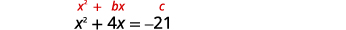The variable terms are on the left side. Take half of $$4$$ and square it.$$\left(\frac{1}{2}(4)\right)^{2}=4$$ Add $$4$$ to both sides.Factor the perfect square trinomial, writing it as a binomial squared.Use the Square Root Property.Simplifying using complex numbers.Subtract $$2$$ from each side.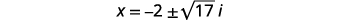Rewrite to show two solutions.We leave the check to you.
##### Exercise $$\PageIndex{5}$$

Solve by completing the square: $$y^{2}-10 y=-35$$.

$$y=5+\sqrt{15} i, y=5-\sqrt{15 i}$$

##### Exercise $$\PageIndex{6}$$

Solve by completing the square: $$z^{2}+8 z=-19$$.

$$z=-4+\sqrt{3} i, z=-4-\sqrt{3} i$$

In the previous example, our solutions were complex numbers. In the next example, the solutions will be irrational numbers.

##### Example $$\PageIndex{4}$$

Solve by completing the square: $$y^{2}-18 y=-6$$.

Solution:The variable terms are on the left side. Take half of $$-18$$ and square it. $$\left(\frac{1}{2}(-18)\right)^{2}=81$$Add $$81$$ to both sides.Factor the perfect square trinomial, writing it as a binomial squared.Use the Square Root Property.Simplify the radical.Solve for $$y$$.Check.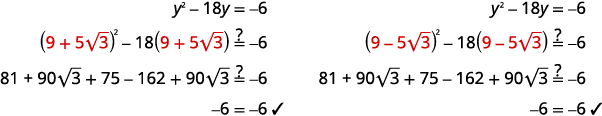Figure 9.2.35Another way to check this would be to use a calculator. Evaluate $$y^{2}−18y$$ for both of the solutions. The answer should be $$−6$$.

##### Exercise $$\PageIndex{7}$$

Solve by completing the square: $$x^{2}-16 x=-16$$.

$$x=8+4 \sqrt{3}, x=8-4 \sqrt{3}$$

##### Exercise $$\PageIndex{8}$$

Solve by completing the square: $$y^{2}+8 y=11$$.

$$y=-4+3 \sqrt{3}, y=-4-3 \sqrt{3}$$

We will start the next example by isolating the variable terms on the left side of the equation.

##### Example $$\PageIndex{5}$$

Solve by completing the square: $$x^{2}+10 x+4=15$$.

Solution:Isolate the variable terms on the left side. Subtract $$4$$ to get the constant terms on the right side.Take half of $$10$$ and square it. $$\left(\frac{1}{2}(10)\right)^{2}=25$$Add $$25$$ to both sides.Factor the perfect square trinomial, writing it as a binomial squared.Use the Square Root Property.Simplify the radical.Solve for $$x$$.Rewrite to show two solutions.Solve the equations.Check:Figure 9.2.47
##### Exercise $$\PageIndex{9}$$

Solve by completing the square: $$a^{2}+4 a+9=30$$.

$$a=-7, a=3$$

##### Exercise $$\PageIndex{10}$$

Solve by completing the square: $$b^{2}+8 b-4=16$$.

$$b=-10, b=2$$

To solve the next equation, we must first collect all the variable terms on the left side of the equation. Then we proceed as we did in the previous examples.

##### Example $$\PageIndex{6}$$

Solve by completing the square: $$n^{2}=3 n+11$$.

Solution:Subtract $$3n$$ to get the variable terms on the left side.Take half of $$-3$$ and square it. $$\left(\frac{1}{2}(-3)\right)^{2}=\frac{9}{4}$$Add $$\frac{9}{4}$$ to both sides.Factor the perfect square trinomial, writing it as a binomial squared.Add the fractions on the right side.Use the Square Root Property.Simplify the radical.Solve for $$n$$.Rewrite to show two solutions.Check: We leave the check for you!
##### Exercise $$\PageIndex{11}$$

Solve by completing the square: $$p^{2}=5 p+9$$.

$$p=\frac{5}{2}+\frac{\sqrt{61}}{2}, p=\frac{5}{2}-\frac{\sqrt{61}}{2}$$

##### Exercise $$\PageIndex{12}$$

Solve by completing the square: $$q^{2}=7 q-3$$.

$$q=\frac{7}{2}+\frac{\sqrt{37}}{2}, q=\frac{7}{2}-\frac{\sqrt{37}}{2}$$

Notice that the left side of the next equation is in factored form. But the right side is not zero. So, we cannot use the Zero Product Property since it says “If $$a⋅b=0$$, then $$a=0$$ or $$b=0$$.” Instead, we multiply the factors and then put the equation into standard form to solve by completing the square.

##### Example $$\PageIndex{7}$$

Solve by completing the square: $$(x-3)(x+5)=9$$.

Solution:We multiple the binomials on the left.Add $$15$$ to isolate the constant terms on the right.Take half of $$2$$ and square it. $$\left(\frac{1}{2} \cdot(2)\right)^{2}=1$$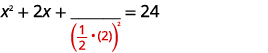Add $$1$$ to both sides.Factor the perfect square trinomial, writing it as a binomial squared.Use the Square Root Property.Solve for $$x$$.Rewrite to show two solutions.Simplify.Check: We leave the check for you!
##### Exercise $$\PageIndex{13}$$

Solve by completing the square: $$(c-2)(c+8)=11$$.

$$c=-9, c=3$$

##### Exercise $$\PageIndex{14}$$

Solve by completing the square: $$(d-7)(d+3)=56$$.

$$d=11, d=-7$$

## Solve Quadratic Equations of the Form $$ax^{2}+bx+c=0$$ by Completing the Square

The process of completing the square works best when the coefficient of $$x^{2}$$ is $$1$$, so the left side of the equation is of the form $$x^{2}+bx+c$$. If the $$x^{2}$$ term has a coefficient other than $$1$$, we take some preliminary steps to make the coefficient equal to $$1$$.

Sometimes the coefficient can be factored from all three terms of the trinomial. This will be our strategy in the next example.

##### Example $$\PageIndex{8}$$

Solve by completing the square: $$3 x^{2}-12 x-15=0$$.

Solution:

To complete the square, we need the coefficient of $$x^{2}$$ to be one. If we factor out the coefficient of $$x^{2}$$ as a common factor, we can continue with solving the equation by completing the square.Factor out the greatest common factor.Divide both sides by $$3$$ to isolate the trinomial with coefficient $$1$$.Simplify.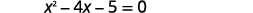Add $$5$$ to get the constant terms on the right side.Take half of $$4$$ and square it. $$\left(\frac{1}{2}(-4)\right)^{2}=4$$Add $$4$$ to both sides.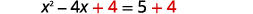Factor the perfect square trinomial, writing it as a binomial squared.Use the Square Root Property.Solve for $$x$$.Rewrite to show two solutions.Simplify.Check:Figure 9.2.80
##### Exercise $$\PageIndex{15}$$

Solve by completing the square: $$2 m^{2}+16 m+14=0$$.

$$m=-7, m=-1$$

##### Exercise $$\PageIndex{16}$$

Solve by completing the square: $$4 n^{2}-24 n-56=8$$.

$$n=-2, n=8$$

To complete the square, the coefficient of the $$x^{2}$$ must be $$1$$. When the leading coefficient is not a factor of all the terms, we will divide both sides of the equation by the leading coefficient! This will give us a fraction for the second coefficient. We have already seen how to complete the square with fractions in this section.

##### Example $$\PageIndex{9}$$

Solve by completing the square: $$2 x^{2}-3 x=20$$.

Solution:

To complete the square we need the coefficient of $$x^{2}$$ to be one. We will divide both sides of the equation by the coefficient of $$x^{2}$$. Then we can continue with solving the equation by completing the square.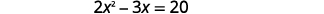Divide both sides by $$2$$ to get the coefficient of $$x^{2}$$ to be $$1$$.Simplify.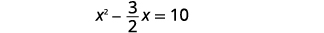Take half of $$-\frac{3}{2}$$ and square it. $$\left(\frac{1}{2}\left(-\frac{3}{2}\right)\right)^{2}=\frac{9}{16}$$Add $$\frac{9}{16}$$ to both sides.Factor the perfect square trinomial, writing it as a binomial squared.Add the fractions on the right side.Use the Square Root Property.Simplify the radical.Solve for $$x$$.Rewrite to show two solutions.Simplify.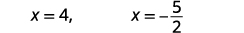Check: We leave the check for you!
##### Exercise $$\PageIndex{17}$$

Solve by completing the square: $$3 r^{2}-2 r=21$$.

$$r=-\frac{7}{3}, r=3$$

##### Exercise $$\PageIndex{18}$$

Solve by completing the square: $$4 t^{2}+2 t=20$$.

$$t=-\frac{5}{2}, t=2$$

Now that we have seen that the coefficient of $$x^{2}$$ must be $$1$$ for us to complete the square, we update our procedure for solving a quadratic equation by completing the square to include equations of the form $$a x^{2}+b x+c=0$$.

##### Howto: Solve a Quadratic Equation of the Form $$a x^{2}+b x+c=0$$ by Completing the Square
1. Divide by aa to make the coefficient of $$x^{2}$$ term $$1$$.
2. Isolate the variable terms on one side and the constant terms on the other.
3. Find $$\left(\frac{1}{2} \cdot b\right)^{2}$$, the number needed to complete the square. Add it to both sides of the equation.
4. Factor the perfect square trinomial, writing it as a binomial squared on the left and simplify by adding the terms on the right
5. Use the Square Root Property.
6. Simplify the radical and then solve the two resulting equations.
7. Check the solutions.
##### Example $$\PageIndex{10}$$

Solve by completing the square: $$3 x^{2}+2 x=4$$.

Solution:

Again, our first step will be to make the coefficient of $$x^{2}$$ one. By dividing both sides of the equation by the coefficient of $$x^{2}$$, we can then continue with solving the equation by completing the square.Divide both sides by $$3$$ to make the coefficient of $$x^{2}$$ equal $$1$$.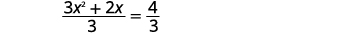Simplify.Take half of $$\frac{2}{3}$$ and square it. $$\left(\frac{1}{2} \cdot \frac{2}{3}\right)^{2}=\frac{1}{9}$$Add $$\frac{1}{9}$$ to both sides.Factor the perfect square trinomial, writing it as a binomial squared.Use the Square Root Property.Simplify the radical.Solve for $$x$$.Rewrite to show two solutions.Check: We leave the check for you!
##### Exercise $$\PageIndex{19}$$

Solve by completing the square: $$4 x^{2}+3 x=2$$.

$$x=-\frac{3}{8}+\frac{\sqrt{41}}{8}, x=-\frac{3}{8}-\frac{\sqrt{41}}{8}$$

##### Exercise $$\PageIndex{20}$$

Solve by completing the square: $$3 y^{2}-10 y=-5$$.

$$y=\frac{5}{3}+\frac{\sqrt{10}}{3}, y=\frac{5}{3}-\frac{\sqrt{10}}{3}$$

Access these online resources for additional instruction and practice with completing the square.

## Key Concepts

• Binomial Squares Pattern
If $$a$$ and $$b$$ are real numbers,
• How to Complete a Square
1. Identify $$b$$, the coefficient of $$x$$.
2. Find $$\left(\frac{1}{2} b\right)^{2}$$, the number to complete the square.
3. Add the $$\left(\frac{1}{2} b\right)^{2}$$ to $$x^{2}+bx$$
4. Rewrite the trinomial as a binomial square
• How to solve a quadratic equation of the form $$a x^{2}+b x+c=0$$ by completing the square.
1. Divide by $$a$$ to make the coefficient of $$x^{2}$$ term $$1$$.
2. Isolate the variable terms on one side and the constant terms on the other.
3. Find $$\left(\frac{1}{2} \cdot b\right)^{2}$$, the number needed to complete the square. Add it to both sides of the equation.
4. Factor the perfect square trinomial, writing it as a binomial squared on the left and simplify by adding the terms on the right.
5. Use the Square Root Property.
6. Simplify the radical and then solve the two resulting equations.
7. Check the solutions.

6.2: Solve Quadratic Equations by Completing the Square is shared under a CC BY license and was authored, remixed, and/or curated by OpenStax.